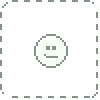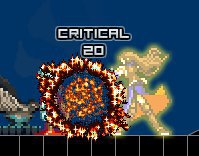## Deviation Actions

3K Views
ENTER = PAUSE
Z = BackStep /
X = L-hand
C = R-hand
Spacebar = Jump
UpArrow = Open Treasure,Active Laver,access depth door

SpaceBar+DownArrow =
1.[on cloud box] = drop fall
2.[on air] = drop kick

Attack Equipment
change Equipment Slot
Key 1 - 9

Special Command
press A then press command and press A again to confirm spells
Command Avaliable
A+[up]+[up]+[up]+A Immune Mode (Damage = 0)
A+[down]+[down]+[down]+A Bravery Mode (no effect now)
A+[up]+[down]+[up]+A Endure Mode (no effect with pushback)
A+[down]+[up]+[down]+A DEBUFF Mode
A+[down]+[up]+[down]+[up]+[down]+A Gravity Cut Mode
A+[left]+[left]+[right]+[right]+A SpeedUp Mode

Spell Command
A+X+X+A Spell fireball
A+C+C+A Spell ice spike
A+[down]+[down]+[up]+A Spell fire wall
Image details
Image size
660x450px 265.14 KB
Published:Impressive work.

what program did u use? is this a finished engine? Where can I get it?couldn't resist but collect all the keys. Didn't know where to go later. Great engine!Cool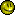I love Castlevania. I think SOTN is my favourite game of all time.thank you for comment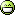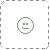I noticed that from your rockman engine to this one, slope functionality was improved. If you don't mind me asking, what was your method for slope correction? i.e. staying on slopes even when moving down them
My own implementation works but is a little bit hacky and your implementation looks like it works quite well.

Looks great so far!thanks for comment// my english is not good ,that why too hard to expland my slopefunction.
my slope function check like this concept.

this my slope check function XD . I hope this will help you more or less .

value
1 is wall(unmoveable)
2 is space(moveable)
3 is cloudBox
4 is water
5 is slope/45degree
6 is \45degree
7 is /(45/2)lower part
8 is /(45/2)upper part
9 is \(45/2)lower part
10 is \(45/2)upper part

function CheckSlope() {
var charPos : Point = new Point(this.x,this.y-1);
var currentMapPos : Point = Coor2map(charPos);
var values:Number = (currentMapData[currentMapPos.y][currentMapPos.x]);

if (values==1 || values==3) {
charPos = new Point(this.x, this.y + (tileSize-1));
currentMapPos = Coor2map(charPos);
values = (currentMapData[currentMapPos.y][currentMapPos.x]);
}

if (values==4) {
this.y = (currentMapPos.y+1)*tileSize-(this.x%tileSize);
} else if (values==5) {
this.y = (currentMapPos.y+1)*tileSize-(tileSize-(this.x%tileSize));
}else if (values == 6) {
this.y = (currentMapPos.y+1)*tileSize-((this.x%tileSize)/2);
}else if (values == 7) {
this.y = (currentMapPos.y+1)*tileSize-((this.x%tileSize)/2)-tileSize/2;
}else if (values == 8) {
this.y = (currentMapPos.y+1)*tileSize-((tileSize/2)-(this.x%tileSize)/2);
}else if (values == 9) {
this.y = (currentMapPos.y+1)*tileSize-((tileSize/2)-(this.x%tileSize)/2)-tileSize/2;
}else if (values==0) {
this.y = -0.001+(currentMapPos.y)*tileSize;
}

charPos = new Point(this.x,this.y);
currentMapPos = Coor2map(charPos);
values = (currentMapData[currentMapPos.y][currentMapPos.x]);
if (values==0) {
this.y = -0.001+(currentMapPos.y)*tileSize;
}

}sorry little mistake. the value is start from 0 to 9
value
0 is wall >> 9 is \(45/2)upper part